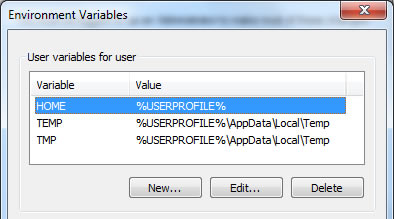#### JavaScript 函数(Currying)柯里化

``` a(3)(4)(4) // 48```

1. 如果固定函数的调用次数

``` const a = function(x) { return function(y) { return function(z) { return x * y * z; }; }; };```

``` const result = x => y => z => x * y * z;```

2. 通用方法

``` function curry(fn) { const len = fn.length; return function curried() { const args = Array.prototype.slice.call(arguments); if (args.length >= len) { return fn.apply(this, args); } return function () { return curried.apply(this, args.concat(Array.prototype.slice.call(arguments))); }; }; }```

``` function curry(fn, ...args){ return args.length === fn.length ? fn(...args) : (...next_args) => curry(fn, ...args, ...next_args); }```

``` const result = curry(function (a, b, c) { return a * b * c; });```

``` const result2 = curry(function (a, b, c) { return a + b + c; });```

``` let x = a(3)(4) let y = x(4); console.log(x); // 12 console.log(y); // 48```

3. 特殊的柯里化

``` function currying(x){ var sum = x var curried = function(y){ sum = sum * y return curried } curried.toString=function(){ return sum } return curried }```

``` console.log(currying(1)) //1 console.log(currying(1)(2)) //2 console.log(currying(1)(2)(3)) //6 console.log(currying(1)(2)(3)(4)) //24```

1. 函数 a 执行之后返回一个函数 b，函数 b 中 的 arguments 是 调用函数 b 时候传入的参数。
2. `Array.prototype.slice.call` 是在类数组上调用数组的 slice 方法，将类数组变为真正的数组，例如调用函数是传入的 arguments 就是一个类数组，他是没有 slice 等数组的方法的，所以使用 `Array.prototype.slice.call` 来将其变为真正的数组。

``` function a(){ return function (){ console.log(arguments); } } var b = a();```

``` function (){ console.log(arguments); }```

``` const a = function (a, b, c) { return a + b + c; } const result2 = curry(a);```

``` result2 = function curried() { const args = Array.prototype.slice.call(arguments); if (args.length >= len) { return fn.apply(this, args); } return function () { return curried.apply(this, args.concat(Array.prototype.slice.call(arguments))); }; }; });```

``` const args = Array.prototype.slice.call(arguments);```

``` result3 = function () { return curried.apply(this, args.concat(Array.prototype.slice.call(arguments))); }```

``` args.concat(Array.prototype.slice.call(arguments))```

``` const args = Array.prototype.slice.call(arguments);```

#### 自定义 Angular 2 的 Pipe(Filter)

``` angular .module('app') .filter('leftPad', function(value, anotherValue){ // do something with `value` and `anotherValue` // and return a value });```

``` <p>{{ num | leftPad:searchValue }}</p>```

``` controller(\$filter) { var numbers = [1,2,3,4,5,6,7,8,9,10,11,12,13,14,15,16,17,18,19,20]; this.numbers = numbers.map(number => \$filter('leftPad')(number, searchValue)); }```

``` // leftPad.pipe.ts import { Pipe, PipeTransform } from '@angular/core'; @Pipe({name: 'leftPad'}) export class LeftPad implements PipeTransform { transform(value: number, anotherValue: number): string { // do something with `value` and `anotherValue` // and return a value } }```

``` <p>{{ num | leftPad:searchValue }}</p>```

``` export class App { constructor(private leftPad: LeftPad) { let numbers = [1,2,3,4,5,6,7,8,9,10,11,12,13,14,15,16,17,18,19,20]; this.numbers = numbers.map(number => this.leftPad.transform(number, searchValue)); } }```

#### 你可能不知道的 NPM VERSION

NPM 现在变得越来越流行了，但是很多人可能只是用了其中一小部分的功能，但是 NPM 其实有一大票功能可以用，不信你试试 npm -help 看看。

``` { "name" : "npm-version", "description" : "npm version command", "version" : "1.1.1611" }```

``` npm version patch```

``` npm version minor```

``` npm version major```

``` "scripts" : { "postversion" : "git push && git push --tags" }```

``` "scripts" : { "start" : "node app.js", "pretest":"rm -Rf build && gulp build && cd build && npm install --production", "test":"./test/run.sh", "preversion":"npm test", "postversion" : "git push && git push --tags" }```

#### Git 每次提交时的用户名和密码设置

2015/09/14 09:25 2 条评论 6315 次浏览

1. 在Windows中添加一个HOME环境变量，值为%USERPROFILE%，如下图：2. 在 “开始>运行” 中打开 %Home%，新建一个名为 _netrc 的文件。

3. 用文本编辑器打开 _netrc 文件，输入 Git 服务器名、用户名、密码，并保存。示例如下：

```machine git.xxx.com
```

OK, 试试吧，还需要不需要用户名密码了。

#### less 检测 是否是某种类型的 函数

2015/05/19 09:41 1 条评论 3190 次浏览

iscolor 是否是颜色 接受正常的颜色关键字和十六位表示法以及RGB表示法等。
isnumber 是否是数字
isstring 是否是字符串
iskeyword 是否是关键字 这个没搞明白到底用来判断什么东西。
isurl 是否是 url  例如 url(…)，貌似必须这样子才行。

ispixel 是否是像素  应该判断给定的值是否是 数字+px 的。
ispercentage 是否是百分比 应该判断给定的值是否是 数字+% 的。
isem 是否是 em
isunit 是否是某个单位的，两个参数 如果待验证的值为指定单位的数字则返回 `true`

.mixin (@a) when (isnumber(@a)) {